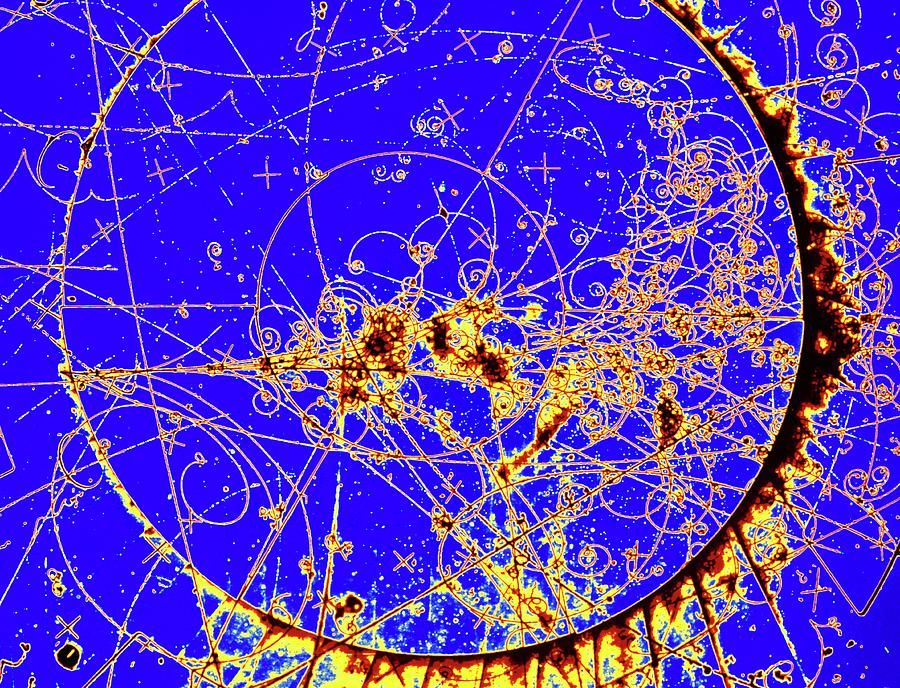# A Primer on Particle Physics, Part 3: Feynman Diagrams

You may have seen physicists draw things like this (before I knew what these were, they always struck me as looking like some kind of esoteric, occult hieroglyphs – the sort of thing you might find a wizened old mage scribbling in his spellbook):This is, in fact, a representation of a process or interaction that particles can undergo, and it’s called a Feynman diagram1. And it is actually an immensely useful tool for actually working with QFT.

Let’s play a game. Here are the rules. You can draw two kinds of lines – a solid line with an arrow pointing in either direction, or a wavy line.You can connect these lines in just one way: three lines can come together at a point, provided those lines are exactly one wavy line, one solid with an arrow pointing toward the point, and one solid with an arrow pointing away from the point. Like this:The game goes like this: I’m going to give you some set of lines on the left and another set of lines on the right (maybe the same, maybe different). Your job is to connect all of these lines by adding stuff in the middle. You can add as many lines and vertices as you want, as long as they obey the rules above. There will, of course, be many possible solutions. We’re not going to care about the length of the lines, or their exact direction – just the overall structure of how they’re connected.

For example, suppose I give you this: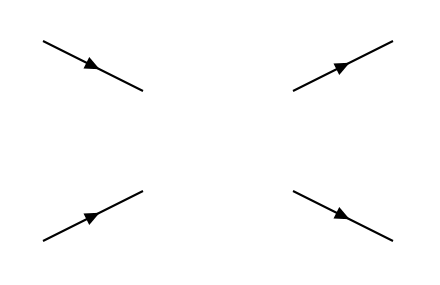A simple solution would be: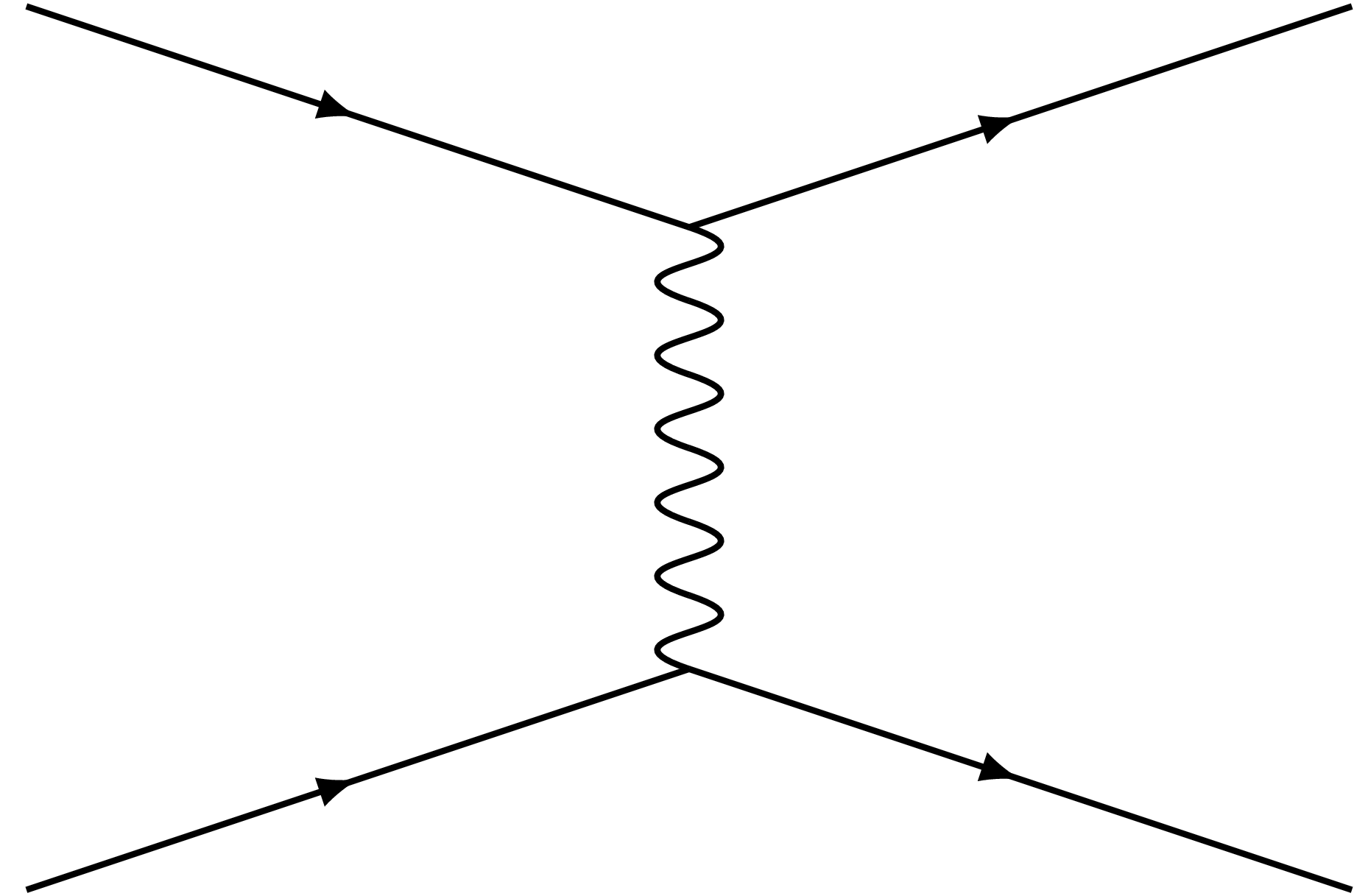Here’s one for you to try. Go ahead, try jotting down some solutions on a piece of paper – remember, you can add as many additional lines in the middle as you like, as long as only these lines connect to the outside. There’s more than one; see how many you can come up with. I’ll wait.Here are the two simplest solutions:

Spoiler[collapse]

But of course, by adding more and more lines and vertices, we can make much more complicated diagrams (in fact, we could make infinitely many):

Spoiler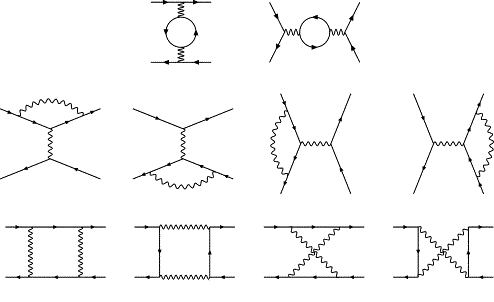[collapse]

So what are we doing? We’re drawing possible processes that can occur in a Quantum Field Theory – in this case, with the specific rules I gave, in Quantum Electrodynamics, or QED.

Each line here represents a particle. Depending on the type of particle, we conventionally draw different sorts of lines – straight lines for fermions and various wavy, curly, or dotted lines for bosons. The horizontal axis is an abstract representation of time, so you “read” this diagram as an interaction happening from left to right. (Some people use the convention where the vertical axis represents time, so their diagrams are rotated ninety degrees, but in my anecdotal experience, most people use the horizontal axis). And each vertex, where multiple lines meet, corresponds to a “coupling” between different particles that is allowed by the theory. Typically, a vertex must have exactly three lines meeting at it.2

Slightly less intuitive is the role of the arrows on those fermion lines; they indicate what’s called “charge flow” or sometimes (misleadingly, I think) “particle flow”. The important thing to note is that when the arrow is pointing right, i.e. with the direction of time, that fermion line indicates a particle; when it points right, against the direction of time, it represents the corresponding antiparticle. Note that this sort of ties in with the idea I mentioned above, that an antiparticle can in some sense be thought of as a time-reversed version of its corresponding particle.3

The “rules” for the game of drawing these diagrams are given by the particular Quantum Field Theory you’re working with. The fields and couplings of the theory (as expressed in its Lagrangian) tell you what kinds of lines you’re allowed to draw and which lines you’re allowed to connect at vertices. Beyond this, charges must be conserved at each vertex. This means, for one thing, that if a line with an arrow comes in to a vertex, a corresponding line with an arrow must come out of that vertex (and if you look at the diagram above, you should be able to satisfy yourself that this condition is satisifed). There also may be other “charges” associated with the particles in a theory that, like electric charge, must be conserved – for instance, in the Standard Model, every particle has a “lepton number” associated with it, and this lepton number must always be unchanged by any interaction. In other words, the “rules” of the Standard Model only allow Feynman diagrams where the total charge and lepton number (and so forth) of all the particles going in is the same as the total coming out.

Finally, and slightly less intuitively, energy and momentum must be conserved – or rather, since the energies and momenta of the particles in the diagram are not specified, it must be possible to assign energies and momenta in a way that conserves them. But note that this really only applies to the lines that have external connections. This is because particles are allowed to violate the usual mass/energy/momentum relations as long as they only appear internally and don’t “exit” the diagram. (These particles that occur solely internally are called “virtual particles”, which I also think is something of a misleading name.)

These diagrams, to be clear, are not just a pictorial tool. Each diagram corresponds to a precise mathematical expression for the “amplitude” of the given process, and there are rules (which, like anything, can be read off from the Lagrangian for the theory) that tell you how to translate those lines and vertices into an expression for the amplitude. The amplitude, in turn, goes into any calculation of the probability for such a process occurring.

Some QED Processes

At the risk of repeating myself, let’s get a tiny bit more concrete and talk about some Feynman diagrams within the field theory of QED, specifically the simplest version of QED, where we have only electrons (and, of course, positrons, their antiparticle) and photons. There is one kind of coupling allowed in QED: a coupling between the electron and the photon. This means that the only kind of vertex allowed is one that has both a wavy line (representing the photon field) and a solid line (representing the electron field). And since charge must be conserved, we have to have the same number of arrows on those solid lines coming in to the vertex as going out. If you think about these rules for a minute, you’ll see that this means that the only vertex that works is the one I described at the beginning of this article, with one arrow coming in, one going out, and one wavy line.

Let’s look at an example: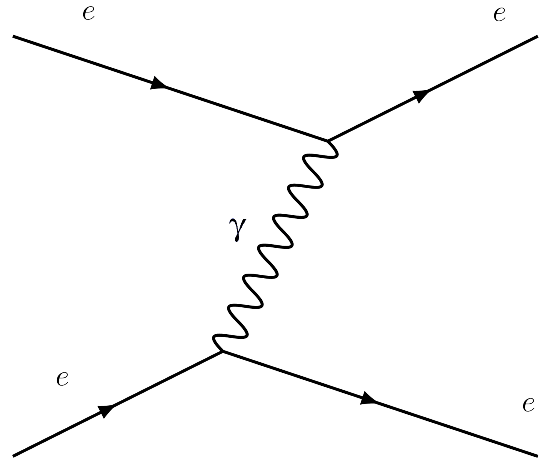What does this represent? Well, remember we read the diagram from left to right. So, we start with two electrons. They’re electrons, not positrons, because the arrows on those lines are pointing forward in time. The Feynman diagram doesn’t say anything about what those electrons are doing; they could each be moving in any direction, with any momentum. They needn’t even necessarily be close to each other, though when you do the math, you find that the probability of this interaction happening decreases the further apart the electrons are. At any rate, as we move along in time, we come to the first vertex – here, we have a solid line with an arrow going in, and then two lines coming out – one a wavy photon line (the$\gamma$ is the symbol physicists use for a photon), the other another solid line, with the arrow still pointing forward in time. In other words, we start with one electron and we end up with an electron and a photon. So this represents an electron emitting a photon – transferring some of its energy into the photon field and starting an oscillation there. As we continue across the diagram, we see that that photon ends in another vertex, with the other electron. This represents the photon being absorbed by the second electron.

The end result is that we started with two electrons and ended with two electrons, but the energies and momenta of the electrons have changed. This constitutes an interaction between the two electrons. In fact, this is what physicists call a “collision” or “scattering”. It’s much as if the electrons were billiard balls – when billiard balls collide, they each start with some energy and momentum, they hit each other (i.e. interact), and then they each move off with some different energy and momentum. And that’s just what has happened with these electrons. Granted, the electrons didn’t actually “hit” each other, in the literal sense of touching. But remember what I said about the distance between the electrons – when you do the math, the probability of this process occurring drops the farther apart the electrons are. So as two electrons approach each other, the probability of this kind of “collision” happening goes up.

And you can do more; using the rules of QED, you can calculate not just a generic probability for this process, but also a probability for the two electrons each to end up with a given momentum after the collision. And if you do that, you find that on average the results you get are the same as if you calculated what would happen using classical electrodynamics. In other words, all these little exchanges of photons amount, in the end, to what we think of as the repulsive force between two negatively charged particles.

As a brief aside, note that we could also switch which electron emits and which absorbs the photon: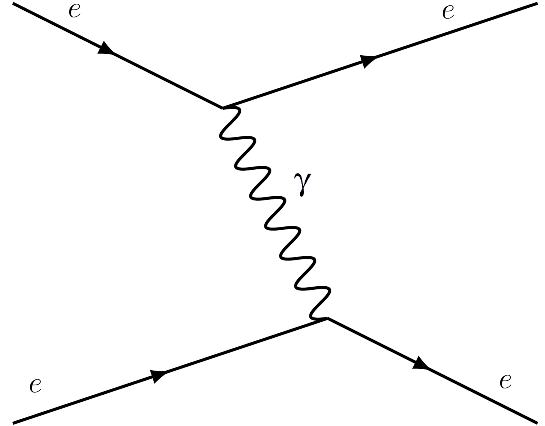In QFT, we actually don’t care which electron emits and which absorbs the photon. (In fact, there are many cases where whether a vertex constitutes emission or absorption depends on what relativistic frame of reference we are in). These don’t actually constitute different diagrams, then, so we typically draw it this way: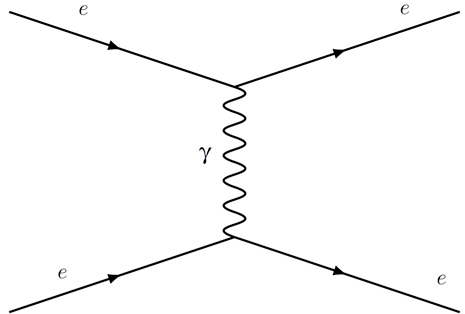Let’s look at a similar but slightly different diagram, one of the “solutions” from the game we played earlier: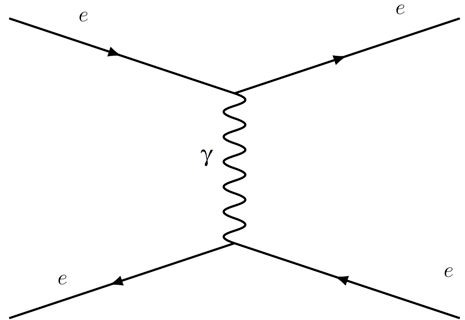This, obviously, looks the same as the one above, except that the arrows on one of the fermion lines are reversed. When the arrows are pointing opposite the direction of time, remember, that means it’s an antiparticle, so in this case we are starting out with an electron and a positron (which, remember, is the name for an antielectron). This diagram, then, represents an electron emitting a photon, which is then absorbed by a positron – or the other way around, a positron emitting a photon, which is then absorbed by an electron.

Here’s a neat thing about QFT, though – there was nothing in the “rules” for making these diagrams that cared about the orientation of the lines. That means that any diagram that obeys the rules of the theory still obeys the rules if we rotate it by ninety degrees. So the following is also a valid diagram:Here, we again start with an electron and a positron, but this time they meet and annihilate into a photon, which then converts back into an electron and positron. Notice that we started and ended with the same particles (an electron and a positron) as in the previous example – all that has changed is what goes on in between. And remember that all that we can actually observe are the particles that we start with and the particles that we end with. So if we were observing these particles – say, shooting an electron and a positron toward each other and seeing what angles they bounce off of each other at – we wouldn’t be able to tell which of those two processes had occurred. Instead, what we’d care about is the total of the probability of the two processes. Physicists call these two different diagrams different “channels” for a single interaction.

And this is a general rule. What we’re really interested in calculating, typically, is: given some initial particles with such and such energy and momentum, what is the total probability of ending up with some other set of particles (possibly the same, possibly different) with some other energy and momentum? To do that, in principle, we must write down all the possible diagrams that start with and end with the right particles, calculate the probability associated with each one, and then sum them all up.

However, there’s a catch – it turns out that for any process, there is actually an infinite number of possible diagrams have the same initial and final sets of particles. We could, after all, add in as much extra stuff in the middle as we like, as long as we don’t break any rules, and still get a valid diagram for the same initial and final particles. For instance, the one-loop solutions I mentioned above would also contribute to the electron/positron interaction:Fortunately, in practice we don’t need to sum up an infinite series of diagrams. I referred earlier to the fact that the diagrams represent mathematical expressions that are used to calculate the probabilities of interactions happening, but I didn’t say anything about how to actually translate the diagrams into numbers. Well, one of the rules for that calculation is that the for each vertex, the probability gets multiplied by a “coupling constant” that represents the strength of the interaction between the particles involved. In most cases4 that coupling constant is significantly less than 1, meaning that for each extra vertex in the diagram, that diagram’s probability gets smaller and smaller.

That means that we can get a decent approximation of the probability for an interaction just by considering the simplest diagrams – the one with the fewest vertices. And if we want to get a more accurate result, we can start adding in more complicated ones. If the coupling constant of the theory is small (and for QED, it is), we can reach levels of precision well beyond the resolution of any experimental apparatus in this way. The more precise we want to get, the more complicated the calculation becomes, but a complicated calculation is much easier than an infinite one. This method of getting increasingly better and better approximations, up to whatever level we want, is called perturbation theory.5

Lest you think that QED is only about electrons and positrons attracting and repelling each other, let’s look at one more: electron-positron annihilation. As I mentioned in the last article, you’ve probably heard about how when antimatter meets normal matter, it annihilates into energy. Here’s one of the processes by which that happens, where we start with an electron and its antiparticle, a positron, and end up with just two photons: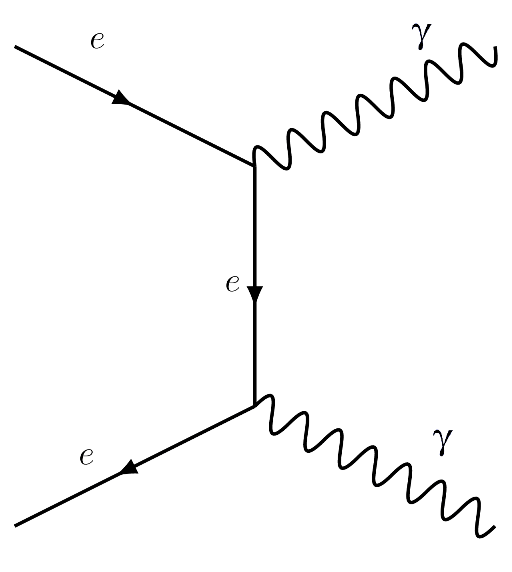Forbidden Processes and Virtual Particles

If I give you arbitrary sets of initial and final particles, there’s no guarantee that there are any allowable Feynman Diagrams that will take you from one to the other. A process for which no valid diagram exists, within a given theory, is called forbidden. Physicists spend a lot of time trying to detect supposedly forbidden processes, because if such a process is observed to happen, that is a smoking gun saying that the theory forbidding it is not correct, or not complete.

If a process is forbidden, this is generally because it can’t occur without violating some conservation law of the theory. For instance, in QED and in the Standard Model, electric charge is conserved. This means that there is no way to draw a valid diagram that, say, starts with two electrons and ends with one electron. And indeed, there’s no way to construct such a diagram out of the lines and vertices we have to work with in QED.

Sometimes, it’s less obvious that a process is forbidden. For instance, could you start with an electron and a positron and end with just one photon? In other words, would the basic vertex that we have in QED be allowable as a diagram on its own?The answer turns out to be “no”. The reason for this is that momentum and energy have to be conserved – the total energy and the total momentum of the particles that come out have to be equal to that of the particles we started with. For a diagram to be valid, then, there has to be at least some combination of energies and momenta you can assign to the initial and final particles such that the totals are conserved. But it turns out to be impossible to do that in the case of the above diagram6. That’s why electron-positron annihilation requires the kind of diagram we saw above, with two photons, rather than just one, in the final statae.

But wait a minute, you might be saying, if it’s impossible to balance energy and momentum across that simple vertex, why is that vertex allowed in diagrams at all? This points to a subtlety that I mentioned above, but will repeat now: energy and momentum have to be conserved between the initial and final particles, but not necessarily at each vertex within the diagram. In other words, the particles that appear only at intermediate stages of the diagram (like the photon in our simple electron/positron scattering diagrams) are allowed to violate the usual equation relating mass, momentum, and energy.

These energy conservation-violating particles are called “virtual particles” – which I think is a bit of a misleading name; I wouldn’t say they’re particularly less “real” than anything else. These virtual particles are given license to exist, so to speak, by the Uncertainty Principle. In addition to the momentum/position uncertainty relation, Heisenberg’s famous principle also puts a limit on the precision with which energy and time can be simultaneously defined. So virtual particles are allowed to deviate from the amount of energy they “should” have, as long as they exist for a short enough time. The bigger the deviation in energy, the shorter the time they’re allowed.

In fact, when you come right down to it, there’s no sharp distinction between which particles count as “virtual” and which as “real”. Any particle can violate the mass/energy relation, as long as it does so within the limits prescribed by the Uncertainy Principle – the longer they exist, the smaller those deviations are allowed to be. What we call “real” particles, then, are just particles that have existed long enough that their deviations from energy conservation are negligible.

Let’s circle back to forbidden processes for a moment to make one more point. What about a process involving two particles that don’t couple to each other? Suppose you have a QFT that includes, among others, a particle A and a particle B, and that there is no interaction term between those two fields in the Lagrangian – in other words, the A and B fields are not coupled. This means that no vertices containing both an A and B particle are allowable in Feynman diagrams. Does that mean that any process involving these two particles (an A and a B scattering off of each other, for instance, or an A converting into a B, or vice versa) is disallowed? Not necessarily – such processes could still be allowed if there is some third particle C that couples to both A and B. There could be no direct interaction between A and B, but there could still be indirect interactions. Any diagrams for such interactions would have to have more vertices, though, since at the very least you’d need an A-C vertex and a a C-B vertex. And because for every additional vertex in the diagram, you decrease the probability associated with the diagram, this kind of indirect interaction will happen much less frequently than it would if it were direct. Processes like these are called suppressed or sometimes semi-forbidden7.

Collisions and Decays

I’ve talked in a general way about using Feynman diagrams to calculate probabilities for things, but in practice there are two different kinds of “things” we could want to calculate probabilities for, and for pragmatic reasons we tend to think and talk about them somewhat differently. First, there are collisions. These are events like the electron/positron interactions I talked about above; we start with two or more particles, they interact, and some other particles (possibly the same, possibly different from the ones we started with) come out. I’ve told you that among the consequences of this kind of process are what we perceive as attractive and repulsive electrical forces; but when we’re interested in studying those fundamental processes themselves, the way we do this is generally by shooting one particle at another and then detecting the particles that come out of the interaction. This is why we build particle colliders. When physicists calculate the likelihood of some particular interaction occurring, they generally talk about it not in terms of probabilities, per se, but in terms of “cross sections”, which might seem a bit counterintuitive, but fundamentally what the question we’re interested in is “If we shoot this particle at that target, what are the chances that they’ll undergo this particular interaction?”8

The other kind of process is what we call a decay. This just means a process in which we start with just one particle. Here’s an example of a diagram for such a process:Don’t worry about the details of this now – this is a process that involves particles and interactions I haven’t talked about yet. Just note that the initial state has just one particle in it, in this case a neutron. What this is saying is that there’s a neutron, just zipping along, minding its own business, and then some stuff happens and we end up, instead of a neutron, having a proton, an electron, and an antineutrino. We say that the neutron has decayed. Note that this does not mean that the neutron was made of a proton, an electron, and an antineutrino. What it means is that the neutron field had an excitation in it, and that excitation was transferred into other fields – some to the proton field, some to the electron field, and some to the neutrino field.9

The fact that this is a valid Feynman diagram in the Standard Model means that if you have a neutron just sitting there, there’s some chance at any time that it could spontaneously decay into a proton, electron, and antineutrino. We could use the Feynman diagrams to calculate a probability per second that this will happen, but the usual way to talk about this is to flip it around and talk about the average lifetime, or half-life10 of the neutron. The bigger the probability of a particular decay happening, the shorter that lifetime will be. So even though there’s no fundamental difference between Feynman diagrams for collisions and for decays, (the only difference is whether you start with one particle or more than one), we use different units to measure their probabilities.

Phase space

All right, this article has gone pretty long, so I’m going to wrap up soon. But I wanted to mention one more concept relating to the processes – interactions and decays – that we’ve been talking about.

We’ve seen that there are, in general, multiple ways that a process can occur. For one thing, there are always multiple “channels” – that is, multiple different diagrams that have the same initial and final sets of particles. Even if we just consider one diagram, though, there are different “options”. Remember that I said one of the rules for Feynman diagrams is that momentum has to be conserved – that is, the total momentum of the final particles has to equal the total momentum of the original particles. But as long as energy is also conserved, that momentum could be divided up between the final particles any way you like. Thus, the process not only has different options in terms of which diagram it follows (i.e. what the intermediate steps are between the initial and final situations), it also has options in terms of how the momentum of the initial particles gets divided up among the final particles.

Consider the neutron decay that we looked at above. Remember two things: energy is conserved, and mass is a form of energy. We start with a neutron at rest, so the total momentum is zero, and all the energy is in the form of the neutron’s mass. In particle physics, we measure energies (and masses) in units of eV, or “electron volts”. The neutron’s mass is about 939.6 million electron volts, or 939.6 MeV. The particles we end up with are a proton, with a mass of 938.3 MeV, an electron, with a mass of 0.5 MeV, and an antineutrino, with a tiny mass of less than 1 eV. If we add these up, we find that the total is about 938.8 MeV, slightly less than the mass of the neutron we started with. Where does that extra 0.8 MeV of energy go? It goes into the kinetic energy of these particles – the proton, electron, and antineutrino fly apart. That 0.8 MeV can be divided up among the three particles in any way, and they can move off in any directions, as long as they do so symmetrically, in the sense that their momenta still balance out to zero. But with only 0.8 MeV to work with, the range of possible ways to divvy up that energy – the available phase space for the decay – is fairly small. If the mass of the neutron were higher, there’d be more excess energy to work with, and the phase space would be larger.

This is an important concept because as a general rule, the more phase space that’s available, the more likely a process is to occur. In other words, it’s easier for a decay or interaction to happen if it has more options for how it happens. This means that very massive particles, which have a lot of mass-energy and therefore a lot of options for how to decay, tend to do so rather quickly. Usually, the higher the mass of a particle, the shorter its lifetime. This also means that when we try to create particles in a particle collider, the more energy we put in, the easier it is. This is why, in our quest to detect heavier and heavier particles, we’ve had to build bigger and bigger colliders. It’ll be good to keep this in mind when we start exploring the particle zoo of the Standard Model next week; remember that the particles we know about right now are the particles we’ve been able to create and detect – for all we know, there could be plenty of other fields/particles in the universe that we don’t know about simply because we haven’t (yet) been able to reach high enough energies to create them.

So, next time we’ll explore the Standard Model and talk about leptons and quarks and stuff.  I’m aiming to get it done and posted next Tuesday, so we’ll see how that goes.